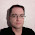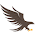## Wednesday, July 20, 2016

### Using MathJax in Blogger

I have added MathJax support to this blog so that I can typeset mathematics using a large subset of $$\LaTeX$$ commands. This functionality works in comments too, which has the nice side effect that it can be used to work around Blogger's limited formatting. In particular, formatting source code in comments can be done as
$$\verb|int foo(void)| \\ \verb|{| \\ \verb| return 0;| \\ \verb|}| \\$$

which is rendered with most of the formatting intact:
$$\verb|int foo(void)| \\ \verb|{| \\ \verb| return 0;| \\ \verb|}|$$

One annoying thing is that the $$\LaTeX$$ commands are not processed when writing or previewing the comment, but they are rendered correctly when it is published...

#### How to enable MathJax in Blogger

MathJax needs to be loaded and configured when the page is loaded. This is done by adding the following
<script type="text/javascript" async="async"
src="https://cdnjs.cloudflare.com/ajax/libs/mathjax/2.7.1/MathJax.js?config=TeX-AMS_CHTML,Safe">
</script>

in the <head> block by editing the blog's template (choose "Template", and click the "Edit HTML" button). This only adds the support to the desktop template, so you need to enable it separately for mobile by pressing the gear button, and choosing the "custom" mobile template (that generates a mobile template from your desktop template).

There are several configurations to choose between, with support for $$\LaTeX$$, MathML, and AsciiMath notation, and control over how much functionality is loaded up front, and how much is loaded on-demand. I have chosen the TeX-AMS_CHTML which enables all $$\LaTeX$$ support but avoids MathML and AsciiMath.

Note the ,Safe modifier added after the configuration name. This disables unsafe constructs such as running javascript from $$\LaTeX$$ commands
$E \href{javascript:alert("Einstein says so!")}{=} mc^2$

This is needed in order to prevent commenters messing up the blog by adding evil constructs in comments.

#### Testing the functionality

Below are some random formulas, just to verify that the rendering works as intended:
$\sigma = \sqrt{\frac{1}{N}\sum_{i=1}^{N}(x_{i}-\mu)^{2}}$
\left\{ \begin{aligned} a_1x+b_1y+c_1z &=d_1+e_1 \\ a_2x+b_2y&=d_2 \\ a_3x+b_3y+c_3z &=d_3 \end{aligned} \right.
$\require{AMScd} \begin{CD} \pi(X, x_{0}) @>\phi_{*}>> \pi(Y, \phi(x_{0}))\\ @VVuV @VVvV\\ \pi(X, x_{1}) @>\phi_{*}>> \pi(Y, \phi(x_{1})) \end{CD}$

The post was updated 2017-04-20 with a new link to fetch MathJax.js as the old MathJax CDN is shutting down.
The post was updated 2017-05-12 to use the bugfixed 2.7.1MathJax release.

#### 15 comments:

1.Testing that $$\LaTeX$$ commands actually work in comments.

Here is the formatted code from the blog post
$$\verb|int foo(void)| \\ \verb|{| \\ \verb| return 0;| \\ \verb|}| \\$$

2.Is there a way to insert pipe (|) char in source code with \verb? Thanks!

1.Yes, the first character after $$\verb!\verb!$$ is the delimiter character, so using e.g. $$\verb+\verb!text!+$$ will let you use the pipe character within the "text".

$$\LaTeX$$ lets you use any character except $$\verb!(!$$ and $$\verb!*!$$ for the delimiter. It is possible that MathJax is slightly more limited, as it is embedded in HTML — but "most" delimiters should work...

3.Thank You ... It works ... (:

4.What if a post doesn't use any Latex? Is there a way to make it so the script isn't downloaded uselessly for that one?

1.I don't know any good way of doing that.

But the MathJax.js is relatively small, and is only loading the rest of the support if it is needed, so the cost of always loading it is not that bad...

5.I see, thank you for your reply.

6.OK, I'm frustrated. I put the code in the <head> element as described, even changed 2.7.0 to 2.7.1 as suggested on the mathjax site, tried removing the async parameter, and nothing works. I even deleted my test blog site from 5 years ago and created a new one. The math isn't being rendered at all.

I have no trouble with MathJax on my own Wordpress site, but I'd like to get away from self-hosting and use blogger if possible.

What am I missing?

1.I'm no blogger expert, so I have no idea... :(

So my only suggestion is to save the source for your and my blog (as rendered by the browser), and try to see if there are any obvious differences...

7.Very thanks, now I will not have to use online Tex exporters to image when I will want to post formula on my blog. However I have chosen to use asciimath over tex, because my math symbol set is tiny, so it is not reasonable to use full-scale solution

8.HOW DO YOU ADD ASCIIMathML.js

9.Unfortunately on my blogspot blog MathJax text goes small on mobile phone and I can't find how to fix it... ��

10.$E \href{javascript:alert("Einstein says so!")}{=} mc^2$

11.$P(E) = {n \choose k} p^k (1-p)^{n-k}$

the dollar sign is replaced by \[ ]\

12.http://holdenweb.blogspot.com/2011/11/blogging-mathematics.html

This page helps